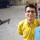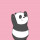1. Home >
2. Apps >
3. Groups >

# simple algebraic puzzle .....

Question asked by Shailaja Tiwari in #Brainy Puzzles on Sep 18, 2013Shailaja Tiwari · Sep 18, 2013
Rank C1 - EXPERT
Q. xyz+xyz+xyz=zzz
Find the value of xyz?

condition: values of xyz must be same throughout the expression. Posted in: #Brainy PuzzlesAnoop Kumar · Sep 18, 2013
Rank A2 - PRO
x=1
y=8
z=5Abhishek Rawal · Sep 18, 2013
Rank A2 - PRO
ianoop
x=1
y=8
z=5
But that doesn't satisfies the equation.
As per your answer, xyz=40, Hence LHS = 40+40+40 = 120. RHS = zzz = 5^3 = 125.
LHS != RHSAnoop Kumar · Sep 18, 2013
Rank A2 - PRO
185+185+185 = 555 😛Abhishek Rawal · Sep 18, 2013
Rank A2 - PRO
Bloody hell!
I thought question is : x*y*z + x*y*z + x*y*z = z*z*z [^_^]rahul69 · Sep 19, 2013
Abhishek Rawal
Bloody hell!
I thought question is : x*y*z + x*y*z + x*y*z = z*z*z [^_^]
If that is the case, then :
x=3;
y=4;
z=6;
xyz+xyz+xyz=(3*4*6+3*4*6+3*4*6)=216=6*6*6=z*z*z
😏Shailaja Tiwari · Sep 19, 2013
Rank C1 - EXPERT
Wow ,great you're absolutely right @ianoop.But how did you get this answer .I mean the logic if you could share would be of great help .I'd tried solving the question in several ways(including trial and error method too but couldn't get it .)
Such questions are generally asked in BARC's RMO.Abhishek Rawal · Sep 19, 2013
Rank A2 - PRO
@Shailaja Tiwari
3xyz = zzz => xyz = zzz/3
Take value of zzz = 111,222,333 upto 999.
Only one will satisfy the condition & that is 555.
Hence 555/3 = 185
So, x=1, y=8 & z=5Anoop Kumar · Sep 19, 2013
Rank A2 - PRO
I started from 3 digit number multiplied by 3 = zzz
start from unit place.
3*z should have z at the unit place.
start from possibilities 3*1=3, 3*6=18 you can come up with 3*5 = 15.
now you got 5 at unit place and carry 1 that means now you need a number with
3*y should have 4 at unit place, so adding a carry 1 will result 5.
and same way you can find value of x.

I hope this way is simple.
May be there Could be other simpler approach.boombastik85 · Oct 21, 2015
Rank E2 - BEGINNER
Rahul 69 is right! xyz is x*y*z, 185 is an interesting answer but does not fit into rules of algebraDivisha Madupalli · Feb 12, 2020
Rank C1 - EXPERT

3z = z or 1z or 2z or 3z and so on

But only z and 1z with satisfy the equation as z has to be between 0 and 9.

=> 3z = z OR 3z = 10 + z

=> z = 0 OR z = 5

=> z=5

Now,

xyz + xyz + xyz = 555

=> xyz = 185

## You must log-in or sign-up to reply to this post.

Click to Log-In or Sign-Up